﻿

### Functional differential inequalities of parabolic type

#### Abstract

We prove a theorem wich generalizes a result of J. Szarski (see ;Theor. 2)concerning weak inequalities fora diagonal system of second order differential functional inequalities of hetype(Error rendering LaTeX formula)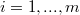$i = 1,...,m$, assuming that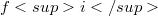$fi$ is parabolic with respect to u for any$i=1,...,m$. After introducing the definition of left parabolic (or right parabolic) function with respect to another one, we obtain the over mentioned generalization (Theor. 2.2) as a consequence of a theorem about strong inequalities (Theor. 2.1) which is a generalization of Theor.1 of  in the case of left parabolic (or right parabolic) functions. These generalizations have been suggested by the following example in wich we have the assertion of Theor. 2 of  even if hypotheses of the theorem are not al1 verified. Consider the function f defined as (l)(Error rendering LaTeX formula) for(Error rendering LaTeX formula) belonging to the set of real and symmetried 2 x 2 matrices and z continuous function in Đ, with continuous in D partial second derivatives with respect to x as well as functions u and v defined assuming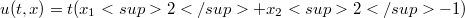$u(t,x) = t (x_{1}2 + x_{2}2 - 1)$ and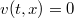$v(t,x) = 0$ for every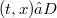$(t,x) ∈ D$.

DOI Code: 10.1285/i15900932v9n2p173

Full Text: PDF